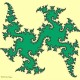#### [SOLVED] Can a subset of the plane have nontrivial $H_2$ or $\pi_2$?

This is a question that occurred to me years ago when I was first learning algebraic topology. I've since learned that it's a somewhat aesthetically displeasing question, but I'm still curious about the answer.

Is it possible for a subset of $\mathbb R^2$ to have a nontrivial singular homology group $H_2$? What about a nontrivial homotopy group $\pi_2$?#### @Ian Agol 2014-12-09 22:20:39

Apparently the asphericity is due to Zastrow (see Cannon-Conner-Zastrow).

Also apparently the result that the higher homology groups vanish is due to Zastrow, but his habilitation thesis never seems to have appeared.#### @j.c. 2018-06-14 17:54:04

Thanks for finding the link to Zastrow's revised paper. I found a page of his which links to both the old and new versions and briefly describes the differences mat.ug.edu.pl/~zastrow/Nnonano.htm . Versions of his Habilitationsschrift are also available here homepage.ruhr-uni-bochum.de/Andreas.Zastrow/pitriv.htm#### @Jeff Strom 2014-12-09 21:32:45

The higher-dimensional analog has the surprising answer "yes". Namely, for $n\geq 2$, the $n$-dimensional Hawaiian earring $H_n = \bigcup_{k=1}^\infty S(k)$, where $S(k)\subseteq \mathbb{R}^{n+1}$ is the $n$-sphere with center ${1\over 2k}\mathbf{e}_1$ and radius ${1\over 2k}$ has nonzero homology in arbitrarily high dimensions. This is a result of Barratt and Milnor (An Example of Anomalous Singular Homology).